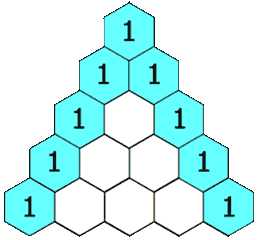## 12.2 Outline

1. Committee problem
1. definition
2. combination formula
3. deck of cards
2. Pascal’s triangle
1. n choose r
2. table entries
3. Counting with the binomial theorem
1. binomial theorem
2. number of subsets

## 12.2 Essential Ideas

Combinations
A combination of r elements selected from a … See the whole entry

## Reference Topics 6-1

This is an elementary introduction to polynomials.
http://www.purplemath.com/modules/polydefs.htm

This is an elementary introduction to multiplying polynomials.
http://www.purplemath.com/modules/polymult.htm

This first part of this site provides some practice polynomial terminology, and with working with polynomials. It has some nice links.

This … See the whole entry

## 6.1 Outline

1. Terminology
1. term
2. polynomial
1. monomial
2. binomial
3. trinomial
3. degree
1. of a term
2. of a polynomial
3. linear
4. numerical coefficient
2. Simplification
1. like terms/similar terms
2. simplify – this is the first of four main processes in algebra
3. Shortcuts with products
1. FOIL (see
See the whole entry

## Individual Research Projects Section 4.5

### Project 4.7

Build a working model of Napier’s rods.

### Project 4.8

Write a paper and prepare a classroom demonstration on the use of an abacus.
Build your own device as a project.

### Project 4.9

Write a paper regarding the invention … See the whole entry

## Reference Topics 1-1

PASCAL’S TRIANGLE:
An interactive version of Pascal’s Triangle.
http://mathforum.org/dr.cgi/pascal.cgi

Here is another site on Pascal’s triangle:

PROBLEM SOLVING:
This site give a nice introduction to problem-solving.
http://mathfocus.tripod.com/page3.html
A site which lists other sites on mathematical puzzles:
https://www.mynhusd.org/See the whole entry

Videos:

Questions to ask yourself to help with your success in mathematics (time: 4:18)

Problem solving strategies (time 3:10)

Creativity and Problem Solving (time: 8:57)

Here is a short clip showing how to build Pascal’s triangle:???See the whole entry

## Homework Hints 1-1

### Note: Homework Hints are given only for the Level 1 and Level 2 problems.

However, as you go through the book be sure you look at all the examples in the text. If you need hints for the Level 3 … See the whole entry

## Section 1.4: Review for Chapter 1

Studying for a chapter examination is a personal process, one which nobody else can do for you. Simply take the time to review what you have done.

Here are the new terms in Chapter 1.

Addition law of exponents [1.3]… See the whole entry